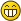Operational iterationXorterFellowPosts: 93 Threads: 30 Joined: Aug 2016 07/01/2017, 12:31 PM Hi, everyone! I have been looking for the real meaning of iteration but without any success. First of all, let us clarify, what we mean under iteration. Well, in english (not like in some european languages like hungarian) there are two meanings of iteration: operationally and functionally. These ones differ to each other in much. (F o F)(x) = F^o2 (x), this is functionally iteration and composition. (I suggest people to call it "repetition", because it is not THE iteration.) x + x + ... + x = x*y, this is (operationally) iteration which is the key to solve the problem of continouesly of Hyper function... so what is between addition and multiplication and so on? If we iterate addition, we get multiplication. If we iterate multiplication, we get power. If we iterate power, we get tetration, then pentation, hexation, heptation etc. . If we iterate composition, we get functional power... If we iterate derivation, we get derivative power... and so on... But what is it? What is the method of the iteration? What is the formula of it? Let us make the situation concrete. What is between the addition and multiplication? Now let us sign them like these ones: +(x):=2+x and *(x)=2*x=2x, let A(x) be equal to H(2;1.5;x)=2[1.5]x (x+y)/2 >= sqrt(xy) >= ssqrt(x^y) >= ... Thus I suppose that: +(x) < A(x) < *(x) if x>2 We might be sure that A(2) = 2[for every x]2 = 4 Okey, but what is the half-iteration of addition? And usually, what is the iteration? Xorter UnizoXorterFellowPosts: 93 Threads: 30 Joined: Aug 2016 07/03/2017, 07:47 PM In my view, the composition and iteration are in very friendly relationship... why? Because Let O be an iteratable operator, let y be a constant and x be a variable. Thus y O y O ... O y = (y O x)^o(x-1) o N = y O^It x If O is addition, O^It is multiplication and so on. But what is N? Well, N is the so-called Neutral number for the operator O, which can be 0 for addition, 1 for multiplication and its iterateds, x for composition, 1 for functional power and its iterateds etc... So it can be a formula for iteration, but how to repeat ("iterate")? It is iterated iteration...or the correct expression: repeated iteration. I am interested in this equation: ( (y O x)^o(x-1) o N )^z = y O^(Iterated z times) x How can we use it? And am I right with these expressions? Xorter Unizotommy1729Ultimate FellowPosts: 1,906 Threads: 409 Joined: Feb 2009 07/27/2017, 12:24 AM (07/01/2017, 12:31 PM)Xorter Wrote: Hi, everyone! I have been looking for the real meaning of iteration but without any success. First of all, let us clarify, what we mean under iteration. Well, in english (not like in some european languages like hungarian) there are two meanings of iteration: operationally and functionally. These ones differ to each other in much. (F o F)(x) = F^o2 (x), this is functionally iteration and composition. (I suggest people to call it "repetition", because it is not THE iteration.) x + x + ... + x = x*y, this is (operationally) iteration which is the key to solve the problem of continouesly of Hyper function... so what is between addition and multiplication and so on? If we iterate addition, we get multiplication. If we iterate multiplication, we get power. If we iterate power, we get tetration, then pentation, hexation, heptation etc. . If we iterate composition, we get functional power... If we iterate derivation, we get derivative power... and so on... But what is it? What is the method of the iteration? What is the formula of it? Let us make the situation concrete. What is between the addition and multiplication? Now let us sign them like these ones: +(x):=2+x and *(x)=2*x=2x, let A(x) be equal to H(2;1.5;x)=2[1.5]x (x+y)/2 >= sqrt(xy) >= ssqrt(x^y) >= ... Thus I suppose that: +(x) < A(x) < *(x) if x>2 We might be sure that A(2) = 2[for every x]2 = 4 Okey, but what is the half-iteration of addition? And usually, what is the iteration? What you are looking for has already been answered by me. To give a short answer  ( this occured in " zeration , hyperoperator , generalized distributive property etc , those kind of keywords ) The solution is b = sqrt(2) f(x,y,z) = ln_b ^[z] ( exp_b ^[z](x) + exp_b^[z](y) ) Notice b < eta. Hence fixpoint methods apply ! Think about it b4 you ask a new question. It really works ! Regards Tommy1729 Return of the master « Next Oldest | Next Newest »

 Possibly Related Threads… Thread Author Replies Views Last PostHyper-Operational Salad Numbers Catullus 9 2,281 09/17/2022, 01:15 AM Last Post: CatullusWeak Hyper-Operational Etas and Euler Numbers Catullus 0 627 06/17/2022, 09:45 AM Last Post: Catullus

Users browsing this thread: 1 Guest(s)